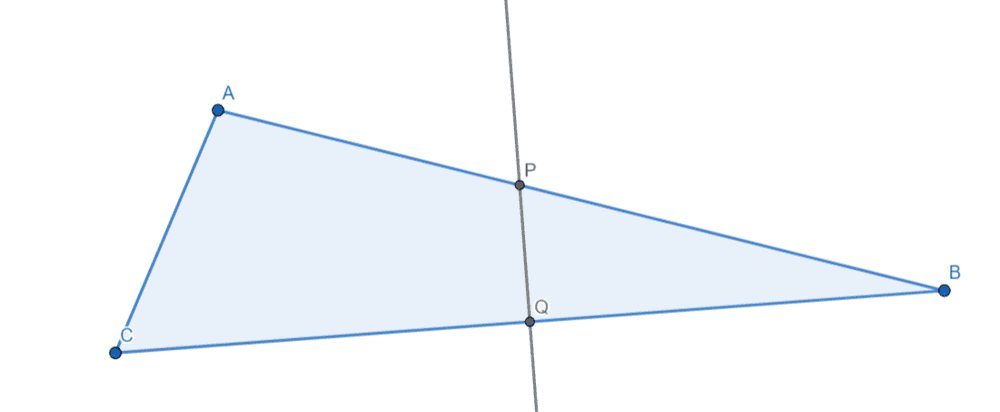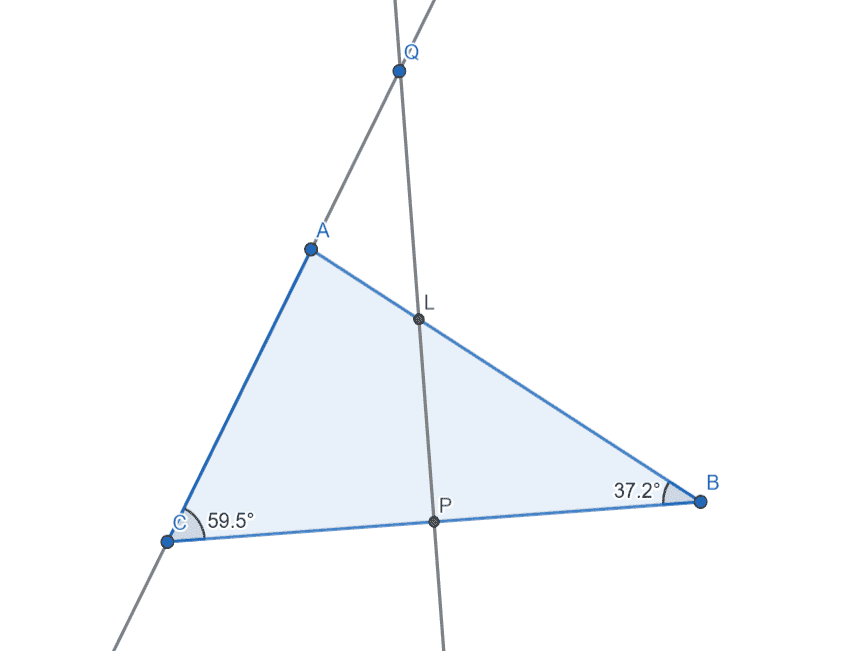# Perpendicular Bisector of a triangle

Homework Statement:
##ABC## is a triangle such that ##\angle ABC = 37^{\circ}15'##, ##\angle ACB = 59^{\circ}40'##, ##BC = 8## cm; the perpendicular bisector of ##BC## cuts ##BA##, ##CA## produced at ##P, \ Q##. Find the length of ##PQ##.
Relevant Equations:
##\tan(\alpha) = \frac{opposite \ side}{adjacent \ side}##
Here is my attempt to draw a diagram for this problem:I'm confused about the "the perpendicular bisector of ##BC## cuts ##BA##, ##CA## produced at ##P, \ Q##" part of the problem.
How does perpendicular bisector of ##BC## cut the side ##CA##?

mjc123
Homework Helper
It cuts CA produced. Extend the line CA until it meets the bisector. That point is Q (not where you have put it).

•nmnna and FactChecker
It cuts CA produced. Extend the line CA until it meets the bisector. That point is Q (not where you have put it).
Thank you

It cuts CA produced. Extend the line CA until it meets the bisector. That point is Q (not where you have put it).I changed my diagram.
Now I have the right triangle ##\triangle PQC##, where ##CP = 4##cm (since ##PQ## is a perpendicular bisector), ##\angle QCP = 59^{\circ}40'##, so I can find ##PQ## using the relation $$\tan\angle QCP = \frac{PQ}{CP}$$
I got ##\approx 6.818## which is not the answer given in my textbook. Where did I go wrong?

Last edited:
Lnewqban
Gold Member
View attachment 280723
I changed my diagram.
Now I have the right triangle ##\triangle PQC##, where ##CP = 4##cm (since ##PQ## is a perpendicular bisector), ##\angle QCP = 59^{\circ}40'##, so I can find ##PQ## using the relation $$\tan\angle QCP = \frac{PQ}{CP}$$
I got ##\approx 6.818## which is not the answer given in my textbook. Where did I go wrong?
The description seems confusing to me as well.
Could it be that point P should be located where the perpendicular bisector of BC cuts BA?
If it is close to 3.75 cm, then your last diagram is not correct regarding location of P.

•nmnna
mjc123
•